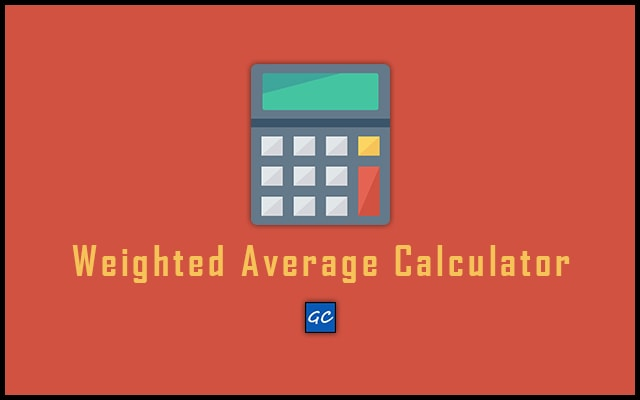# Weighted Average Calculator – Weighted Mean Calculator

In the Weighted Average Calculator, you can find the average value of courses. Also, it is known as the Weighted mean calculator.

 Weight Grade(%) Weighted Average: Total of Weights: Full Calculation:
Related#### Weighted Average Calculation

The calculation or mechanism behind this tool is very simple. See the formula and definition below.

Defination: It’s a sum of each grade(G) multiple by credit/weight(W) and then divide whole result by total number of credits/weights(W).

Formula:

Weighted Average =
G1 * W1 + G2 * W2 + G3 * W3 + … + Gn * Wn
W1 + W2 + W3 + … + Wn
##### Example

Now let’s take an example to get more ideas.

Assume that there are three courses for that we have to find the weighted average.

The values are as below:

1) Quizzes have 90 numbers of grade and its weight is 30.
2) Mid Exam Paper has grade 85 and weight 20.
3) The physics course has grade 65 and weight 40.

In table format it looks like this:

Quizzes 90 30
Mid Exam 85 20
Physics 65 40

The final calculation is:

(30 * 90 + 20 * 85 + 40 * 65)
(30 + 20 + 40)
= 77.77777778

## How to use Weighted Average Calculator?

Follow the steps below to use this online tool.

• First of all open the Weighted Average Calculator in any browser.
• After opne the calculator, you can see there are many input fields.
• The first column of input fields are to enter the weight.
• And the second one is to enter Grade values(in %)
• So, enter the values as per your need and click on “Calculate” button.
• As a result, you will get the Weighted Average, Total of Weights, and Full Calculation below. That’s it.
• In addition, you can use “Reset” button for recalculation. Also, you can click on “Add Row” button to add more courses.

### Conclusion

Above all discuss was about Weighted Mean Calculator. You can use this tool and get average values very quickly. Also, the design is very user-friendly. So, people can use it very easily on desktop and mobile devices. In addition, this tool gives you 100% accurate results. Because it works on pre-defined algorithms. Therefore, no possibilities for the wrong output.